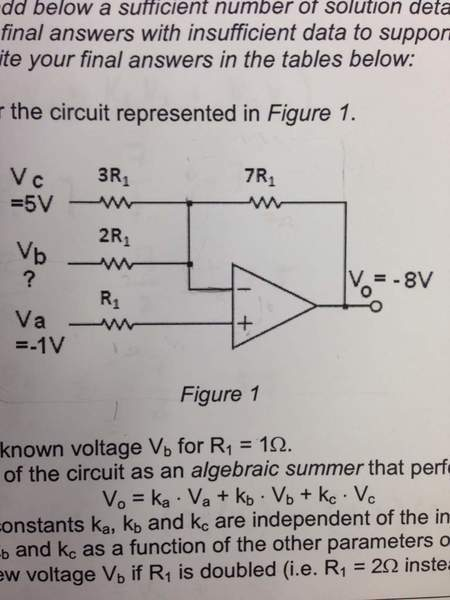Op amp circuit analysis

• Engineering
I have this problem where it's asking for the unknown voltage V(b). What information I get is what is in the image provided and that R1= 1 ohm.

Okay so as I start this problem I remember that the voltage drop across the terminals is pretty much equal to zero because the current flowing through the terminals is zero.

So what I'm thinking is that there will not be any current in resistor R1 because it is attached to the terminals and there is no current through the terminals. This means that the voltage at the non-inverting terminal will be equal to -1V and it will be the same at the inverting terminal (because of the no voltage across the terminals). Here's where I get confused (IF I'm still right up until this point).

I'm confused on where the current is coming from and where it is going but This is how I thought the problem would be solved. Would I use KCL equations from current flowing out of resistors 2R1 and 3R1 at the node to the right of 2R1 to find the voltage V(b) ? I keep thinking that the feedback loop (wire with resistor 7R1) needs to be included in this but am not totally sure how to go about this.

If I'm on the right track then that is awesome! Thanks for anyone's help before hand!donpacino
Gold Member
You are correct. Do a kcl at the negative terminal of the op amp.

That makes me so happy to hear that I'm actually on the right track with this for once! Hahah

I'll try it out and get back to here in a bit. Thank you!

AlephZero
Homework Helper
Okay so as I start this problem I remember that the voltage drop across the terminals is pretty much equal to zero because the current flowing through the terminals is zero.

That's not quite right. There is no voltage difference between the input terminals, and no current flowing into them, for an ideal op-amp.

Current can flow into or out of the output terminal.

That's not quite right. There is no voltage difference between the input terminals, and no current flowing into them, for an ideal op-amp.

Current can flow into or out of the output terminal.

Okay thank you for that correction, I also kind of neglected the output terminal for this because I thought it wouldn't be needed to find the voltage at the second resistor